﻿
Reach Us+44-1202068036
Privacy-preservation Framework for Sharing Genomic Data: A Game Theoretic Approach

# Journal of Health & Medical InformaticsOpen Access

Like us on:

All submissions of the EM system will be redirected to Online Manuscript Submission System. Authors are requested to submit articles directly to Online Manuscript Submission System of respective journal.

# Privacy-preservation Framework for Sharing Genomic Data: A Game Theoretic Approach

Alese BK1 and Adebayo OT2*
1Department of Cyber Security Science, Federal University of Technology, Akure, Nigeria
2Department of Information Technology, Federal University of Technology, Akure, Nigeria
*Corresponding Author: Adebayo OT, Department of Information Technology, Federal University of Technology Akure, PMP 704, Akure, Nigeria, Email: [email protected]

Received Date: Oct 12, 2018 / Accepted Date: Oct 22, 2018 / Published Date: Nov 11, 2018

### Abstract

Sharing genomic is important for healthcare but privacy must be protected because links between de-identified genomic data and named persons can be re-established by users with malicious intents. In this paper, a game theoretic approach is developed for quantifiable protections of genomic data sharing. This approach accounts for adversarial behavior and capabilities. The game model is developed to discover the best solution for sharing genomic summary statistics under an economically driven recipient’s (adversary) inference attack based on a Stackelberg game. The inference attack checks if a targeted DNA is in a genome pool with published summary statistics (that is, minor allele frequency of Single Nucleic Polymorphisms).

Keywords: Game; SNP; MAF; Genomics; DNA; DUA

#### Introduction

This is huge information encoded in the human genome and these are very useful in personalized medicine, paternity testing, disease susceptibility testing and genetic compatibility testing. The question on everybody’s mind is; how can we protect patient privacy while still making the most out of their data?  Some researchers are apprehensive that preservation of privacy might be impossible to realize, even when sharing only summary statistics . This concern is obsessed with high profile demonstrations over the past decade of how de-identified genomic data can be tracked back to named persons, leading to public apologies .

A model for genomic data dissemination can be achieved using game theory to account for adversarial behavior and capabilities. The proposed approach is unique about genomic data privacy, though such techniques have already been used to analyse the reidentification risk in .

In this paper, game model is applied to determine the optimal set of protections for genomic data sharing using a public resource, the Sequence and Phenotype Integration Exchange (SPHINX) system for a case study. SPHINX reports single-nucleotide polymorphism (SNP) summary statistics (that is, MAFs) on data collected from the NIH-sponsored Electronic Medical Records and Genomics Pharmacogenomics (eMERGE-PGx) network .

#### Related Works

In this section, a brief overview of existing techniques adopting cryptographic and non-cryptographic approaches is presented. Cryptographic approaches make computation on encrypted data to guarantee the privacy of individuals. A cryptographic approach to outsource genomic sequences in a cloud server is presented [5,9]. Researchers leveraged a trusted hardware inside untrusted cloud to ensure privacy [5-7]. This secure hardware helps server to execute queries independently. Instead of homomorphic encryption, the authors use symmetric cryptosystem. However, both of these techniques can only process count queries. Some recent works from scientists shows some secure versions of statistical algorithms used in genomic studies like Hardy-Weinberg Equilibrium, Pearson Goodness-Of-Fit Test, and Linkage Disequilibrium . A new notion of ‘Similar Patient Queries’ was introduced by  which showed the importance of secure ranked query. The main contribution of this work approximated Edit Distance which can be securely computed between two parties. With this distance (or string difference) you can rank the sequences of different patients and get similar patients like the searched one. There are a number of other cryptographic solutions proposed for genomic data privacy. These techniques use either homomorphic encryption Yao’s garbled circuit or both as the underlying secure computation primitive.

Non-cryptographic approaches implement various sanitization techniques to ensure the privacy of genomic data. Privacy preserving data publishing (PPDP) has been researched extensively for various types of data. These techniques study how to transform raw data into a version that is immunized against privacy attacks but that still preserves useful information for data analysis. Existing techniques are primarily based on two major privacy models: k anonymity and ε-differential privacy. In spite of its wide applicability in the healthcare domain, recent research results indicate that k anonymity-based techniques are vulnerable to an adversary’s background knowledge. This has stimulated a discussion in the research community in favor of the ε-differential privacy model, which provides provable privacy guarantees independent of an adversary’s background knowledge. However, it is not well understood yet whether differential privacy is the right privacy model for biomedical data as it fails to provide adequate data utility proved that de-identification is an ineffective way to protect the privacy of participants in genome-wide association studies [3,4].

Building upon  and  provide quantitative guidelines for researchers willing to make a certain number of SNPs publicly available in GWAS, without revealing the presence of a single individual within a study group. Scientist proposed using differential privacy to protect the identities of participants in scientific study . In the same vein, researchers proposed privacy-preserving algorithms for computing various statistics related to the SNPs, while guaranteeing differential privacy [9,10]. Scientist proposed privacy-preserving schemes for medical tests and personalized medicine methods that use patients’ genomic data . For privacy-preserving clinical genomics, a group of researchers proposes to outsource some costly computations to a public cloud or semi-trusted service provider .

#### Methodology

Genome contains very sensitive information about the owners. Predisposition to some diseases can be determined based on Single Nucleotide Polymorphism (SNPs). SNP occurs when a nucleotide at a specific position on the Deoxyribonucleic acid (DNA) varies between individuals of a given population

The SNPs of all individuals are represented by the random variable X that takes value in the set X={0,1,2}^(n × m),containing n individuals and m SNPs in a single DNA sequence. The genomic privacy game is depicted using equation 1.1:

G=({P,S,U} ) (Σ(SNP)) 1.1

where P is the set of players (sharer and recipient), S is the set of strategies, U is the set of payoff functions and Σ is a finite set of (DNA) alphabets Σ={A(Adenine), C(Cytosine), G(Guanine), T(Thiamine)}. Sharer(S) and Recipient (R) are the two actors involved in genomic data sharing interactions. The sharer is an investigator of a study or an organization such as an academic medical center that manages genomic data and the recipient requests and accesses the data for some purpose (For example, replication of published findings or discovery of new associations). The privacy worry is on recipient with potential to exploit named genomes or targets by determining their presence in the research study. A core motivation for both sharing and attacking genomic records is the belief that the data have intrinsic value. The sharer benefits by gaining utility from disseminating data, while the recipient benefits by detecting (and exploiting) the targets. Attacks entail costs, such as obtaining identified data needed for linkage, as well as the human capital or computational power necessary to run the attack. The sharer’s decision about a combination of instituting a DUA and technical protection measures (For instance, suppressing information on certain SNPs), as well as the recipient’s decision as to whether an attack is worth its cost, constitute a Stackelberg game, a natural model of this interaction. In this model, the sharer is a leader who can (1) require a DUA with liquidated damages in the event of a breach of contract and (2) share a subset of SNP summary statistics from a specific study (suppressing the rest). The recipient of the data then follows by determining whether or not the benefits gained by attacking each target outweigh the costs. Importantly, the sharer chooses the policy that optimally balances the anticipated utility and privacy risk. To be precise, g is defined as a set of genomic variants (or SNPs) to be shared, a as a set of individuals to be attacked, B_s (g) as the benefit, and Ĉ_s (a) as the estimated cost to the sharer. The sharer’s goal is to maximize the following payoff function by selecting the best strategy g^*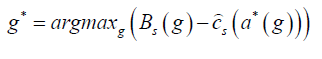1.2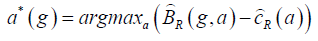1.3

where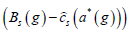represent the sharer’s payoff and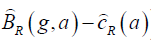is the recipient’s payoff.

In this model, the recipient aims to maximize his or her own payoff (achieved through exploiting the data) and thus determines the subset of targets that they believe can successfully be attacked. We use Ĉ_R (a) to define the estimated cost to the recipient for attacking targets, which includes the expected prefixed liquidated damage penalty for breach of DUA. The estimated benefit to the recipient is denoted B ̂ _R (g,a) which is the benefit the recipient expects to gain from attacking targets.

For any successfully attacked target, the recipient gains a fixed amount, GR, but the sharer loses a fixed amount, LS. As a result, both the sharer’s cost and the recipient’s benefit are proportional to the number of successfully attacked targets.

To simulate the recipient’s uncertainty, the framework introduced by  which compares MAFs between the shared data and a public reference is adopted (which assumes the shared data are drawn from a reference population), so that the estimated number of successfully attacked targets is computed as: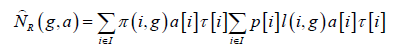1.4

where π(i,g) is the posterior probability that target i is in the study, I is the set of individuals available for attack, p[i] is the prior probability that target i is in the study, l(i,g) is the likelihood ratio comparing the likelihood that target i is in the study versus that it is in a reference population, τ[i] is the probability that individual i is targeted, and a[i] is a binary variable, which is 1 if target i is attacked and 0 otherwise.

System implementation and results

A Genomic Privacy Game Solver is developed to find the best solution for sharing genomic summary statistics (MAF of SNPs) under an economically motivated recipient(adversary)’s inference attack based on a Stackelberg game model. Inference attack is to infer if a targeted DNA is in a genome pool with published summary statistics (that is, minor allele frequency of SNPs).

MATrix LABoratory (MATLAB) (R2016a)) (9.0.0.341360) is used for modelling, computation, visualization (graphs), data analyses and algorithm development.

In Experimental Setup, the proposed model is applied to determine the optimal set of protections for genomic data sharing. The model is evaluated with the data of 8,194 individuals from the Sequence and Phenotype Integration Exchange (SPHINX) system datasets (Auton,2015) for a case study. SPHINX reports single-nucleotide polymorphism (SNP) summary statistics (i.e., minor-allele frequencies [MAFs]) on data collected from the NIH-sponsored Electronic Medical Records and Genomics Pharmacogenomics (eMERGE-PGx) network.

The genomic data include 82 genes identified as important for pharmacogenomics, with 38,112 variant regions (that is, areas of the genome with any type of notable variation across individuals), including 51,826 SNPs, and the phenomic data include various clinical factors extracted from the electronic medical records of these participants (that is., diagnosis codes, procedural codes, and medications). SPHINX publishes all summary statistics and requires an end user licensing agreement that prohibits re-identification attempts.

#### Experimental Setup

Datasets

We use the GWAS datasets from the 2015 iDASH Healthcare Privacy Protection challenge, which consists of 311 SNPs located on human chromosome 2 from 200 PGP participants.

Searching for the Best Solution to the Game

Globally and locally optimal solutions to the Stackelberg game are provided via a backward induction algorithm (BIA).

Modules

Algorithm 1.1: Filtering module

[f, λ, u, D′] = Filter (D, R,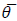, mafcutoff, ldcutoff)

Input: The pool dataset (D), the reference dataset (R), where each row represents an individual, each column represents a SNP, each cell represents the genotype using integer numbers from -1 to 2, where 2 represents minor-minor, 1 represents minor-major, 0 represents majormajor, and -1 represents missing genotype values, the maximal allowable missing rate (), a threshold on minor allele frequency (mafcutoff), and a threshold on the p-value indicating linkage disequilibrium (ldcutoff).

The minor allele frequencies (MAFs) in pool (f), the MAFs in reference (λ), the utilities for each SNP (u), and the remaining pool dataset (D′).

Main body

Handle missing values: For each SNP in the pool dataset (D)

IF the portion of individuals with missing data is smaller or equal to maximal allowable missing rate () remove the SNP from both datasets;

Compute MAFs: For each SNP in the pool dataset (D)

Compute its MAF in pool (f) as the sum of all individuals’ values, divided by number of individuals with no missing data, divided by 2;

For each SNP in the reference dataset (R)

Compute its MAF in reference (λ) as the sum of all individuals’ values, divided by number of individuals with no missing data, divided by 2;

Compute utilities associated with each SNP: For each SNP in the pool dataset (D)

Compute its utility (u) as the absolute difference between its MAF in pool (f) and it’s MAF in reference (λ);

Remove SNPs with MAF smaller than mafcutoff: For each SNP in the pool dataset (D)

IF it’s MAF in pool is smaller than mafcutoff or larger than (1-mafcutoff)

Remove the SNP from both datasets (D, R), the utility vector (u), both MAF vectors (f, λ);Let m be the number of remaining SNPs; Sort the utility vector in descending order and adjust both datasets, both MAF vectors accordingly;

Compute the correlation matrix: For each SNP i from 1 to m

For each SNP j from 1 to m

Compute the Chi-square correlation r2 and corresponding p-value for each SNP-pair;

IF p-value is smaller than ldcutoff

SNP i and SNP j are correlated;

Exclude the SNP outliers: Initialize a Boolean vector Selection of all TRUE values;

Compute the standard deviation of differences between MAF in pool and MAF in reference, σ;

Find ndrop, the number of SNPs that have differences larger than 6σ;

Let the first n drop of the vector be FALSE;

Select a subset of independent SNPs for the sharer: For each SNP i from i to (m-1)

IF Selection (i) is true

For each SNP j from (i+1) to m

IF SNP i and SNP j are correlated

Let Selection (i) be FALSE;

Trim both datasets, both MAF vectors, and the utility vector according to vector Selection;

RETURN f, λ, u, D;

Algorithm 1.2: LR statistics module

LR = Compute_LR (D, f, λ)

Input: The remaining pool dataset (D), the MAFs in pool (f), and the MAFs in reference (f′),

Output: The LR statistics (LR).

Main Body: Let m be the number of remaining SNPs;

Let n be the number of individuals in the pool dataset;

Initialize a n-by-m log-likelihood ratio matrix LR;

For each SNP j from 1 to m

For each individual i from 1 to n

IF D[i, j] == 0

LR[i, j] = 2 * log ((1- f[i])/(1- λ[i]));

ELSE IF [i, j] == 1

LR[i, j] = log ((1- f[i])/(1- λ[i])) + log (f[i]/ λ[i]);

ELSE IF [i, j] == 2

LR[i, j] = 2 * log (f[i]/ λ[i]);

ELSE

LR[i, j]=0;RETURN LR;

Algorithm 1.3: Sharer’s perspective payoff

Ýs=compute_Payoff (LR,u,g,H,GR,ca,cp,Ls,nx)

Input: The LR statistics (LR),the utilities for each SNP (u),the sharer’s strategy (g),the worth of the data to the sharer (H),the prior probability that a target is in the pool (p), the gain to the recipient per successful attack (GR), the cost of access to the recipient per attack (ca), the expected cost of penalty to the recipient per attack (cp), the loss to the sharer per successful attack (LS), and the number of targets (nx).

Output: The sharer’s expected payoff (Ý_s)

Main body: Let m be the number of remaining SNPs;

Let n be the number of individuals in the pool dataset;

Let sum_utility be the sum of the utility vector (u);

Benefit =H*(sum of shared SNPs’ utilities)/sum_utility;

Sum_TP=0;

For each individual i from 1 to n

Sum_LR =0;

For each SNP j from 1 to m

IF SNP j will be shared

Sum_LR=Sum_LR+LR[i, j];

Posterior_prob= exp(Sum_LR)*p;

IF Posterior_prob>1

Psterior_prob = 1;

IF GR*Posterior_prob > (ca + cp)

Sum_TP=Sum_TP+1;

Select_rate=nnxx*p/n;

Cost = LS* Sum_TP* Select_rate;

Ýs= Benefit – Cost;

RETURN Ýs

Algorithm 1.4: Backward induction algorithm

In the Stackelberg game, the sharer needs to evaluate his or her payoff for each available strategy. For each of the sharer’s strategies, the recipient can play any of their own available strategies. The sharer will choose the strategy that maximizes his or her own payoff. Given the large space of possible strategy combinations, BIA is applied to facilitate the search. BIA is a brute force approach that systematically evaluates all of the possible strategies to discover the one with the maximal payoff.

Backward induction algorithm (BIA)

Input: The pool dataset (D),

the reference dataset (R), where each row represents an individual, each column represents a SNP, each cell represents the genotype using integer numbers from -1 to 2, where 2 represents minor-minor, 1 represents minormajor, 0 represents major-major, and -1 represents missing genotype values,the maximal allowable missing rate (),a threshold on minor allele frequency (mafcutoff),a threshold on the p-value indicating linkage disequilibrium (ldcutoff),the worth of the data to the sharer (H),the prior probability that a target is in the pool (p),the gain to the recipient per successful attack (GR),the cost of access to the recipient per attack (ca),the expected cost of penalty to the recipient per attack (cp),the loss to the sharer per successful attack (LS), andthe number of targets (nx).

Output: The sharer’s best strategy (g*), and the sharer’s maximal payoff ( * )

Main Body: [f, λ, u, D′] = Filter (D, R,, mafcutoff, ldcutoff);

LR= Compute_LR (D′, f, λ);

Ý > Ý;

FOR EACH k from 1 to 2m

Let a binary vector g = dec2bin (k);

Ý_S= Compute_Payoff (LR, u, g, H, GR, ca, cp, LS, nx);

IF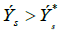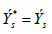g* = g

RETURN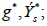#### Experimental Results Presentations

Table 1 shows Average running time (ART) of BIA. n is the number of individuals in the study. m is number of SNPs available for sharing. T is the number of iterations. K is the size of each subpopulation.

m=5
n=20
m=5,
n=200
m=1
n=200
m=15,
n=200
m=20,
n=200
BIA 3 4 4,685 19,831 149,465

Table 1: Average running time (ART) of BIA.

Figure 1 shows a bar chart showing ART of BIA.

Table 2 shows the performance comparison of two algorithms on computational results of the sharer’s payoff. It can be seen that the results of the two algorithms are the same. n is the number of individuals in the study. m is number of SNPs available for sharing.

m=5
n=20
m=5,
n=200
m=15,
n=200
m=15,
n=2000
m=20,
n=200
BIA 755 17,280 16,560 169,020 15,660

Table 2: Computational results (CR).

Figure 2 shows Sharer’s payoff. BIA is compared with the work of researchers that used and Genetic Algorithm which are compared on two types of performance: 1) computational complexity and average running time and 2) accuracy, to determine which one 1) more efficient in terms of computational results.

The parameters used by BIA are shown in Table 3. To measure the runtime (in milliseconds, or ms), we use an Intel Core i7 3 GHz machine, with 8 GB RAM. The average running time is the world clock time averaged across repeated experimental runs. n is the number of individuals in the study.

Parameter Settings
Loss to the sharer per successful attack Ls
The gain to the recipient per successful attack GR
The worth of the data to the sharer H × m
The cost of access to the recipient per attack ca
The expected cost of penalty to the recipient per attack cp
The prior probability that a target is in the study p
The threshold on minor allele frequency Mafcutoff
The threshold on the p-value indicating linkage disequilibrium Ldcutoff
The maximal allowable missing rate ()
The number of targets nx

Table 3: Parameter settings for the backward induction algorithm in the performance analysis.

Table 4 shows the performance comparison of two algorithms on computational complexity and running time with varying size of study dataset. It can be seen that initially, when there are only 5 SNPs and 20 individuals, GA is approximately 263 times slower than BIA. However, as the size of the search space grows, the running time for BIA quickly outpaces GA. By the time there are 20 SNPs and 200 individuals; GA is 189 × faster than BIA. Given that the dataset used in real life scenario case study contains hundreds of SNPs and thousands of individuals. n is the number of individuals in the study. m is number of SNPs available for sharing. T is the number of iterations. K is the size of each subpopulation.

Average running time(ms)
m=5
n=20
m=5,
n=20
m=1,
n=20
m=15,
n=200
m=20,
n=200
BIA 3 4 4,685 19,831 149,465
GA 781 781 783 789 791
BIA/GA 0.0038 0.0051 5.9834 25.1343 188.9570

Table 4: Comparison of algorithms on the computational complexity and the running time.

Table 5 shows the performance comparison of two algorithms on computational results of the sharer’s payoff. n is the number of individuals in the study. m is number of SNPs available for sharing.

 m=5 n=20 m=5, n=200 m=15, n=200 m=15, n=2000 m=20, n=200 BIA 755 17,280 16,560 169,020 15,660 GA 755 17,280 16,560 169,020 15,660

Table 5: Comparison of algorithms on computational results.

#### Conclusion

Biomedical research cannot succeed without human genomic data sharing, and genomic data sharing cannot progress without some reasonable level of assurance that de-identified data from patients and other research participants will stay de-identified after they’re released for research.

Data use agreements that carry penalties for attempted reidentification of participants may be a deterrent, but they’re hardly a guarantee of privacy. Genomic data can be partially suppressed as they’re released; addressing vulnerabilities and rendering individual records unrecognizable, but suppression quickly spoils a data set’s scientific usefulness. Game frameworks provide a quantitative framework for modeling the interaction between sharers and recipients. This game and its solution could serve as a basis for decision making to predict attacker’s behavior. Game theoretical perspective is used to represent the way sharer and recipient can interact with each other around the release of genomic data. Estimating risk and the attacker’s costs, the model estimates the likelihood that any named individual genotype record already held by the attacker is included in the de-identified data set slated for release.

#### References

Citation: Alese BK, Adebayo OT (2018) Privacy-preservation Framework for Sharing Genomic Data: A Game Theoretic Approach. J Health Med Informat 9: 320. DOI: 10.4172/2157-7420.1000320

Copyright: © 2018 Alese BK, et al. This is an open-access article distributed under the terms of the Creative Commons Attribution License, which permits unrestricted use, distribution, and reproduction in any medium, provided the original author and source are credited.

Select your language of interest to view the total content in your interested language

##### Recommended Journals
Viewmore
###### Article Usage
• Total views: 3907
• [From(publication date): 0-0 - Aug 18, 2019]
• Breakdown by view type
• HTML page views: 3852
• PDF downloads: 55

## Post your commentCan't read the image? click here to refresh
###### Peer Reviewed Journals

Make the best use of Scientific Research and information from our 700 + peer reviewed, Open Access Journals

International Conferences 2019-20

Meet Inspiring Speakers and Experts at our 3000+ Global Annual Meetings

Top